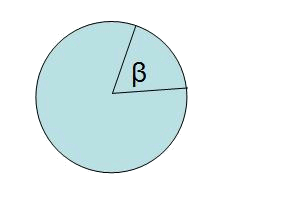# The circleThe perimeter of the circle is: $$P=2\cdot\pi r$$$The area of the circle is: $$A=\pi \cdot r^2$$$

Using proportions it is possible to find the area of a circular sector and the length of the arc: $$\frac{A_{sector}}{A_{cercle}}=\frac{A_{sector}}{\pi \cdot r^2}=\frac{\beta_{degrees}}{360^\circ} \\ \frac{I_{sector}}{perimetre}=\frac{I_{sector}}{2\pi \cdot r}=\frac{\beta_{degrees}}{360^\circ}$$\$

The same proportion can be used with $$\beta$$ in radians and changing $$360^\circ$$ for $$2\pi$$.